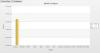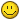## Recommended Posts

Hi All,

I have a Multi-series 2Dcolumn fusion chart. Where x-axis represents months & the y-axis represents number of reports.

If number of reports are less than 5 then values in the y-axis are not showing properly. Please find the attached chart for more details.##### Share on other sitesHi,

Welcome to FusionCharts Forum!Could you please send us the XML code to look into the issue?

##### Share on other sites

Hi,

Actually i am generating xml dynamically using php & mysql.

\$strXML = "<chart caption='Month Vs Report' subCaption='' showBorder='0' formatNumberScale='0' numberSuffix=' Reports' exportEnabled='0' exportAtClient='0' xAxisName='Month' yAxisName='Report' showLegend='1' useRoundEdges='1' animation='1' legendPosition='RIGHT' scrollToEnd='1' zeroPlaneMesh='0'>";

\$strXML .= " <categories>";

for(\$i=1; \$i<=12;\$i++){

\$strXML .= "<category label='".date('F', mktime(0,0,0,\$i,1))."' />";

}

\$strXML .= " </categories>";

\$q= new DBQuery;

foreach(\$result_array as \$result){

\$strXML .="<dataset seriesName='".\$result['year']."'>";

\$reports = getDossierReports(\$_REQUEST['created_by'], \$_REQUEST['shared_with'], \$_REQUEST['program'], \$status, \$start_date, \$end_date, \$view_type, \$result['year'], \$department_user);

\$p=1;

foreach (\$reports as \$total){

for(\$j=\$p; \$j<=\$total['month'];\$j++){

if(\$j==\$total['month']){

\$strXML .="<set value='".\$total['toptal_reports']."' showValue='0' />";

}else{

\$strXML .="<set value='0' showValue='0' />";

}

}

\$p=\$j;

}

for(\$k = \$p ; \$k < 13; \$k++){

\$strXML .="<set value='0' showValue='0' />";

}

\$strXML .= "</dataset>";

}

\$strXML .= "</chart>";

echo renderChartHTML("MSColumn2D.swf", "", \$strXML, "cost", 760, 400, false, true);

Hi,

Welcome to FusionCharts Forum!Could you please send us the XML code to look into the issue?

##### Share on other sites

We are unable to create the XML as it is generating from database.

please send the final XML string which you may get by printing the final \$strXML(the variable containing the XML string in your code) inside any text-area.

##### Share on other sites

Hi,

xml code.

<chart caption='Month Vs Report' subCaption='' showBorder='0' formatNumberScale='0' numberSuffix=' Reports' exportEnabled='1' exportAtClient='0' xAxisName='Month' yAxisName='Report' showLegend='1' useRoundEdges='1' animation='1' legendPosition='RIGHT' scrollToEnd='1' zeroPlaneMesh='0'>

<categories>

<category label='January' />

<category label='February' />

<category label='March' />

<category label='April' />

<category label='May' />

<category label='June' />

<category label='July' />

<category label='August' />

<category label='September' />

<category label='October' />

<category label='November' />

<category label='December' />

</categories>

<dataset seriesName='2010'>

<set value='0' showValue='0' />

<set value='0' showValue='0' />

<set value='0' showValue='0' />

<set value='0' showValue='0' />

<set value='0' showValue='0' />

<set value='0' showValue='0' />

<set value='0' showValue='0' />

<set value='0' showValue='0' />

<set value='0' showValue='0' />

<set value='0' showValue='0' />

<set value='0' showValue='0' />

<set value='0' showValue='0' />

</dataset>

<dataset seriesName='2011'>

<set value='1' showValue='0' />

<set value='0' showValue='0' />

<set value='0' showValue='0' />

<set value='0' showValue='0' />

<set value='0' showValue='0' />

<set value='0' showValue='0' />

<set value='0' showValue='0' />

<set value='0' showValue='0' />

<set value='0' showValue='0' />

<set value='0' showValue='0' />

<set value='0' showValue='0' />

<set value='0' showValue='0' />

</dataset>

</chart>